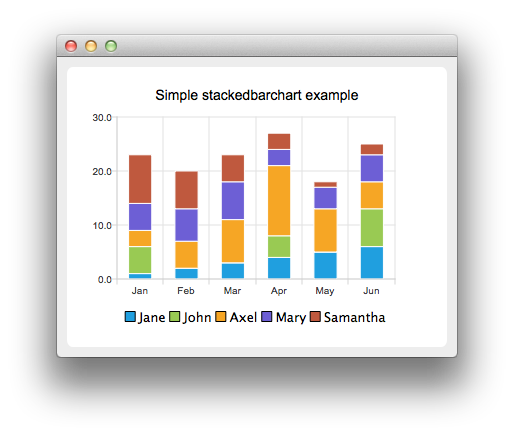# StackedBarChart Example

The example shows how to create a simple stacked bar chart.

The stacked bar chart shows the data in sets as bars stacked on top of each other. The stacking is done per category. The example shows how to create a simple stacked bar chart. Stacked bar chart shows the data in sets as bars that are stacked on top of each other. The stacking is done per category. Creating a stacked bar chart is just like creating a regular bar chart, except that for a stacked bar chart, we use the QStackedBarSeries API instead of QBarSeries.#### Running the Example

To run the example from Qt Creator, open the Welcome mode and select the example from Examples. For more information, visit Building and Running an Example.

#### Creating Stacked Bar Charts

The barsets are used in same way in all barcharts. To illustrate the difference between various barcharts, we use same data in all examples. Data, which the barchart visualizes, is defined by the QBarSet instances. Here we create the sets and append data to them. The data is appended here with << operator. Alternatively the append method could be used.

```    QBarSet *set0 = new QBarSet("Jane");
QBarSet *set1 = new QBarSet("John");
QBarSet *set2 = new QBarSet("Axel");
QBarSet *set3 = new QBarSet("Mary");
QBarSet *set4 = new QBarSet("Samantha");

*set0 << 1 << 2 << 3 << 4 << 5 << 6;
*set1 << 5 << 0 << 0 << 4 << 0 << 7;
*set2 << 3 << 5 << 8 << 13 << 8 << 5;
*set3 << 5 << 6 << 7 << 3 << 4 << 5;
*set4 << 9 << 7 << 5 << 3 << 1 << 2;```

We create the series and append the barsets to it. The series takes ownership of the barsets. The series groups the data from sets to categories. The first values of each set are grouped together in the first category, the second values in the second category etc.

```    QStackedBarSeries *series = new QStackedBarSeries();
series->append(set0);
series->append(set1);
series->append(set2);
series->append(set3);
series->append(set4);```

Here we create the chart object and add the series to it. We set the title for the chart with setTitle, and then turn on animations of the series by calling setAnimationOptions(QChart::SeriesAnimations).

```    QChart *chart = new QChart();
chart->setTitle("Simple stackedbarchart example");
chart->setAnimationOptions(QChart::SeriesAnimations);```

To have the categories displayed on an axis, we need to create a QBarCategoryAxis first. Here we create a category axis with a list of categories and add it to the chart aligned to bottom, acting as the x-axis. The chart takes ownership of the axis. For the y-axis we use a value axis, aligned to the left-hand side.

```    QStringList categories;
categories << "Jan" << "Feb" << "Mar" << "Apr" << "May" << "Jun";
QBarCategoryAxis *axisX = new QBarCategoryAxis();
axisX->append(categories);
series->attachAxis(axisX);
QValueAxis *axisY = new QValueAxis();
series->attachAxis(axisY);```

We also want to show the legend. To do so, we get the legend pointer from the chart and set it to visible. We also place the legend to the bottom of the chart by setting its alignment to Qt::AlignBottom.

```    chart->legend()->setVisible(true);
chart->legend()->setAlignment(Qt::AlignBottom);```

Finally, we add the chart onto a view. We also turn on the antialiasing for the chartView.

```    QChartView *chartView = new QChartView(chart);
chartView->setRenderHint(QPainter::Antialiasing);```

The chart is ready to be shown. We set the chart to be the central widget of the window. We also set the size for the chart window and show it.

```    QMainWindow window;
window.setCentralWidget(chartView);
window.resize(420, 300);
window.show();```

Example project @ code.qt.io